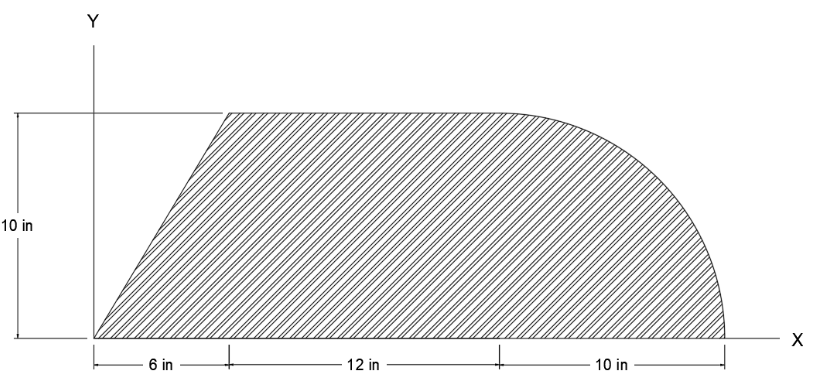# (Solved): a) What is the moment of inertia IY in in4 of the triangular area segment with respect to the y-ax ...a) What is the moment of inertia IY in in4 of the triangular area segment with respect to the y-axis?

b) What is the moment of inertia IY in in4 of the quarter circle with respect to the y-axis?

c) What is the moment of inertia IY in in4 of the composite area with respect to the y-axis?

We have an Answer from Expert

a
moment of inertia of triangular segment about centroidal axis parallel to y-axis
=    =    = 60 in4
area of triangle =   bh =   (6) ( 10 ) = 30
moment of inertia of triangular segment about y-axis
= moment of inertia of triangular segment about centroidal axis parallel to y-axis
+ area ( distance between y-axis and centroidal axis parallel to y-axis )2
= 60 + 30 ( 6 * 2 /3 )2 = 30 ( 42 )
We have an Answer from Expert